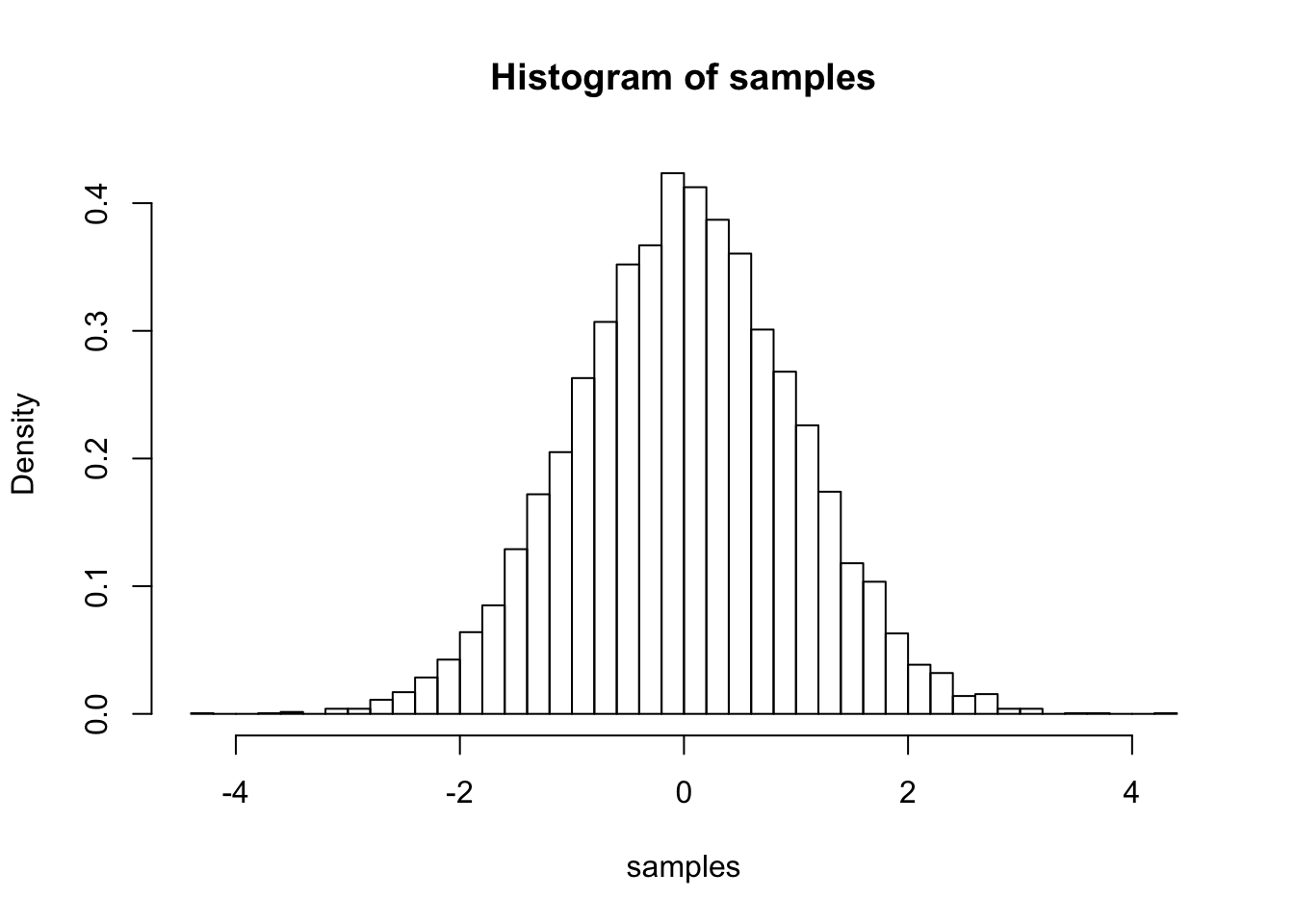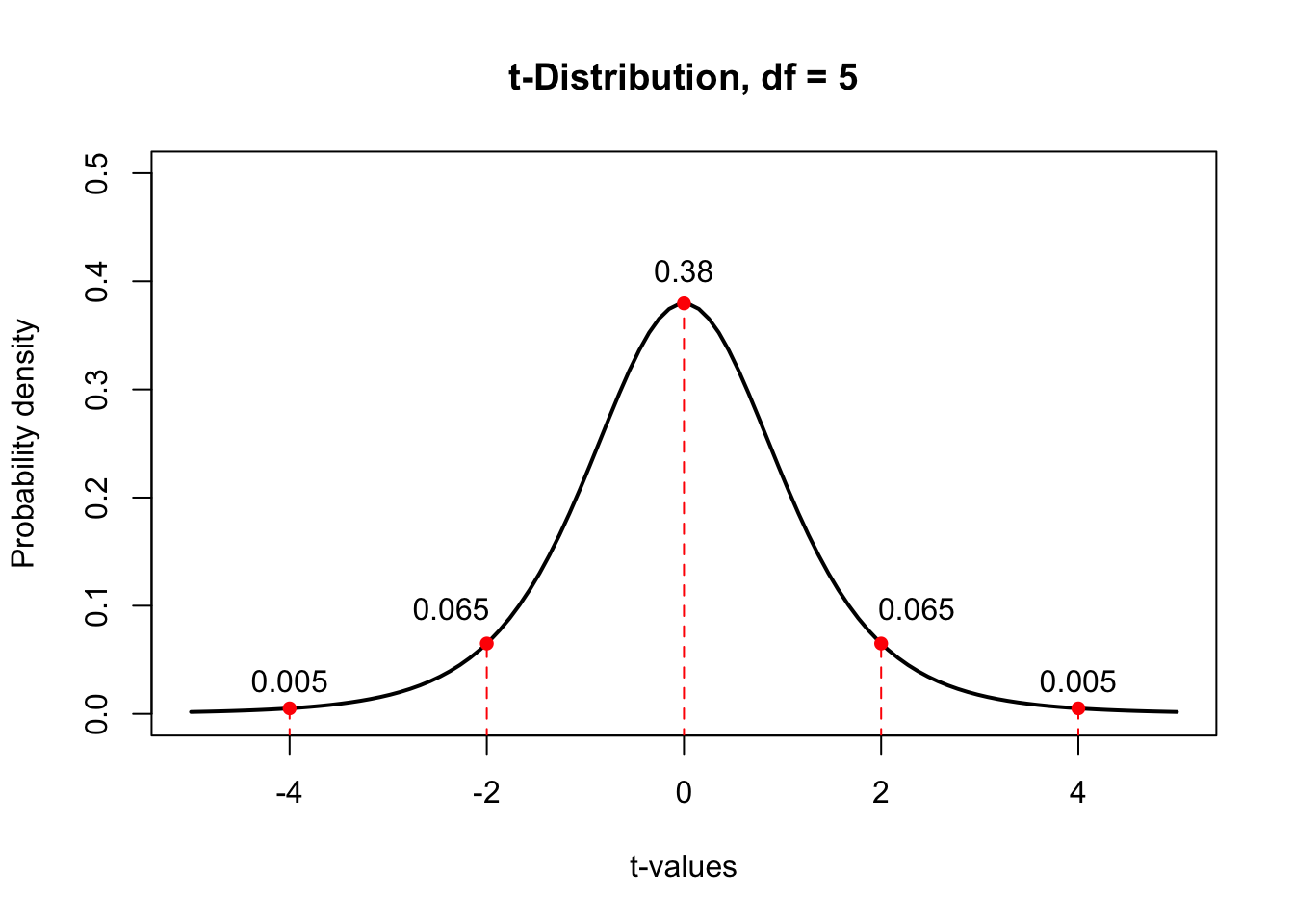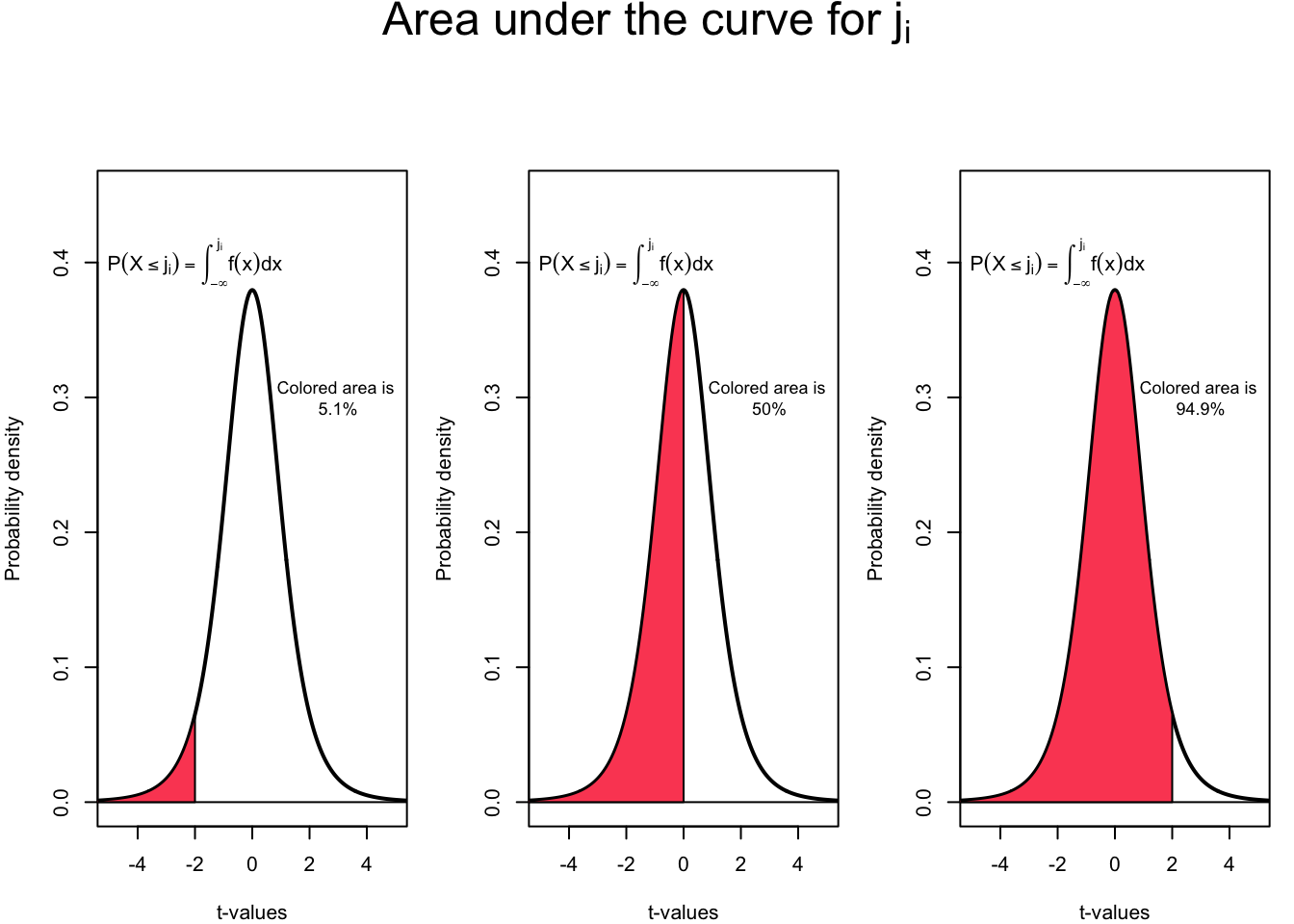The R software provides access to the t-distribution by the dt(), pt(), qt() and rt() functions. Apply the help() function on these functions for further information.

The rt() function generates random deviates of the t-distribution and is written as rt(n, df). We may easily generate n number of random samples. Recall that he number of degrees of freedom for a t-distribution is equal to the sample size minus one, that is,

$df = n - 1\text{.}$

n <- 30
df <- n - 1
rt(n, df)
##    0.73870099  0.72526773  0.57681933 -0.41335675  1.08160178
##   -2.03766753 -0.30897959  1.40177628  1.35433129 -1.14349964
##  -1.01500016  0.25982365  1.06870860 -1.02522571 -0.01872354
##  -1.32623310 -0.53502565 -1.63400367 -0.77143105 -0.49643195
##  -0.32242805 -0.54464546  2.14315143 -2.54128855  0.31696683
##  -0.46055848 -0.26070865 -0.18188416 -0.32236584  0.06151426

Further we may generate a very large number of random samples and plot them as a histogram.

n <- 10000
df <- n - 1
samples <- rt(n, df)
hist(samples, breaks = 'Scott', freq = FALSE)By using the dt() function we may calculate the probability density function, and thus, the vertical distance between the horizontal axis and the t-curve at any point. For the purpose of demonstration we construct a t-distribution with $$df=5$$ and calculate the probability density function at $$t = -4,-2,0,2,4$$.

x <- seq(-4, 4, by = 2)
dt(x, df = 5)
##  0.005123727 0.065090310 0.379606690 0.065090310 0.005123727Another very useful function is the pt() function, which returns the area under the t-curve for any given interval. Let us calculate the area under the curve for the intervals $$j_i = (-\infty, -2], (-\infty, 0], (-\infty, 2]$$ and $$k_i = [-2, \infty),[0, \infty), [2, \infty)$$ for a random variable following a t-distribution with $$df=5$$.

df <- 5
ji <- c(-2,0,2)
pt(ji, df = df, lower.tail = TRUE)
##  0.05096974 0.50000000 0.94903026df <- 5
ki <- c(-2,0,2)
pt(ki, df = df, lower.tail = FALSE)
##  0.94903026 0.50000000 0.05096974